Courses
Courses for Kids
Free study material
Free LIVE classes
More

# JEE Important Chapter - Laws of Motion

Get interactive courses taught by top teachers## Laws of Motion: An Important Concept for JEE

Last updated date: 21st Mar 2023
Total views: 87.9k
Views today: 10

The chapter on laws of motion starts with the basic revision of all the concepts we studied in our lower grades to build the connection between the advanced parts of the laws of motion. In laws of motion, we have discussed Newton's laws of motion, Inertia, derivation of the second law of motion, conservation law, etc….

While deriving the second law of motion we study the relation between the force and momentum, $\vec {F}=\dfrac {d \vec {P}}{dt}$. This relation further leads us to study the conservation of linear momentum which helps us in solving most of the problems related to motion. In the chapter laws of motion, we also studied the force and types of forces along with examples.

We got introduced to a few most important concepts: friction and laws of friction along with the concept of tension. We studied how to determine the forces acting on the body with the help of free body diagrams.

In this chapter students also get to learn about the circular motion where we study an object in circular motion experiencing the different forces (i.e., Centrifugal and Centripetal force). Students will also study the “frames of reference”, a very interesting concept of classical mechanics.

Now, let us move on to the important concepts and formulae related to JEE and JEE main exams along with a few solved examples.

## JEE Main Physics Chapter-wise Solutions 2022-23

### Important Topics of Laws of Motion

• Newton’s Law of Motion Formula

• Linear Momentum

• Conservation of Linear Momentum

• Types of Forces

• Friction and types

• Laws of Friction

• Resultant force

• Tension

• Simple pulley system

### Important Concepts of Laws of Motion

 Name of the Concept Key Points of Concept Newton's Law of Motion Newton's first law gives us a relation between inertia and force. In our day today life we experience Newton's first law and Newton's first law of motion examples.Newton's second law of motion states that force acting on an object is directly proportional to the rate of change of linear momentum.$\vec {F}\propto \dfrac {d \vec P}{dt}$Newton 3 law of motion states that every action is having the equal and opposite reaction.Newton's third law formula is given by:$\vec {F_{12}}=-\vec {F_{21}}$ Linear Momentum and Impulse Linear Momentum is defined as the product of mass and linear velocity, $\vec{P}=m v$Impulse force acting on a body is defined as the product of force and the infinitesimally small interval of time for which it acts,$I=\int_{t_1}^{t_2}\vec {F} dt=\vec {P_2}-\vec{P_1}$ Conservation of Linear Momentum The law of conservation of linear momentum states that “when external forces are not acting, then total momentum of the body remains constant.” In other words, the momentum of the body before impact will be the same as the after impact. Mathematically we write:${m_1}{v_1}={m_2}{v_2}\Rightarrow {v_1}=\dfrac {{m_2}{v_2}}{m_1}$ Concurrent Forces and Equilibrium When a group of forces will be acting on a single point, such forces are known as the concurrent forcesIf the resultant force of these forces is zero, then these concurrent forces are said to be in “equilibrium”. Resultant Force Resultant force is the vector sum of all the forces acting on the body. Friction Friction is the opposition offered when any object is moving a plane.The formula of the friction force is:$\vec {F}=\mu N$Relation between the coefficient of friction and of friction $\theta$:$\theta=\tan^{-1}\mu$ 8. Laws of Friction The total magnitude of limiting frictional force is always proportional to the normal force acting at the contact surface.The magnitude of limiting frictional force is always independent of the total surface area of contact between the surfaces. Tension and Pulley System Tension is defined as a force acting on a stretched string or body. A pulley system is a basic physical apparatus with two or more masses attached to it. 9. Calculation of Tension Acting On a Body The direction of the tension acting on the string is always considered along the string and in a direction opposite to the weight of the body under consideration.$\Rightarrow T=m g$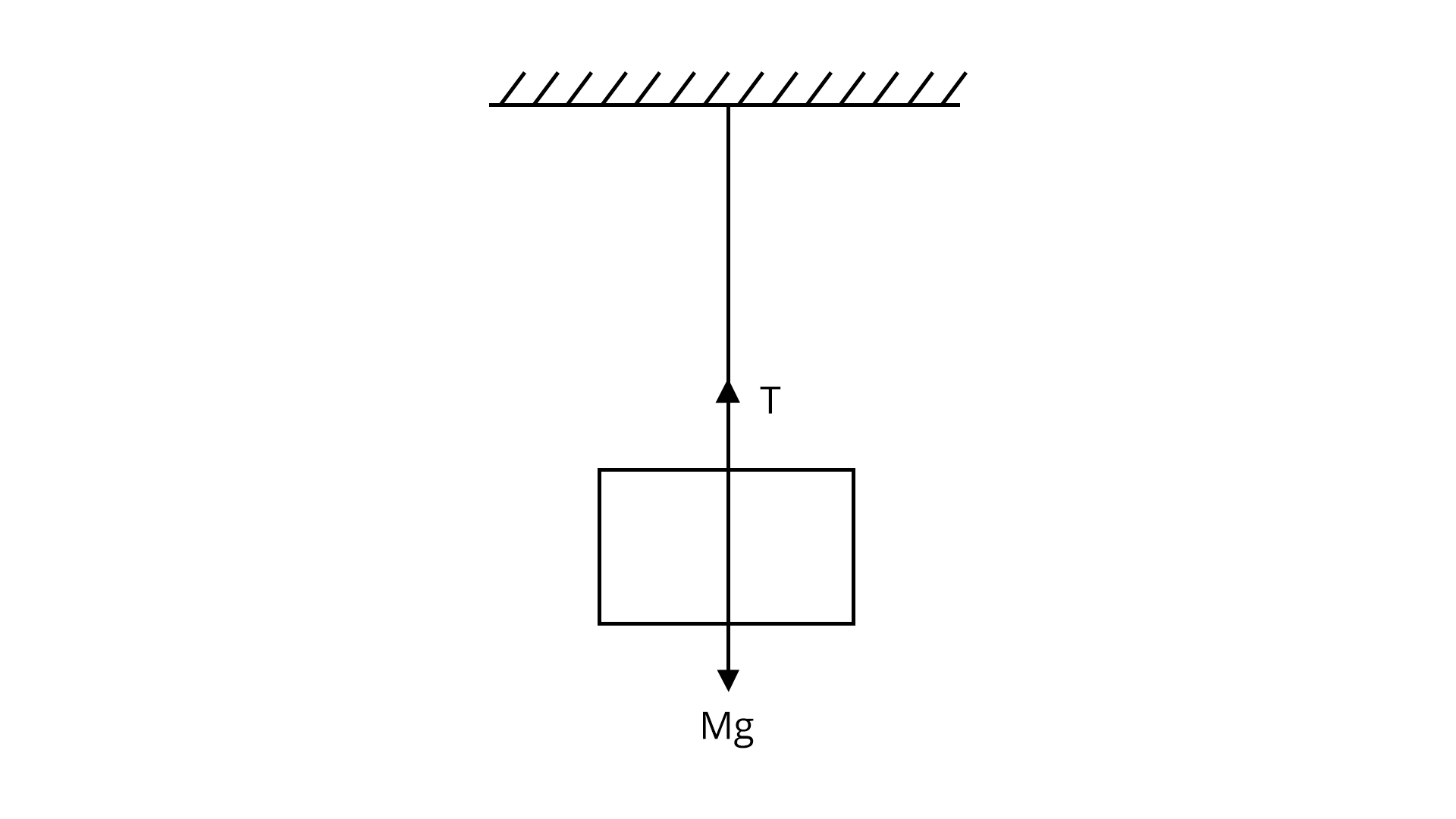Image: A block attached to a string 10. Calculation of Tension In a Simple Pulley The common acceleration ‘a’ acting in a simple pulley system:$a=\dfrac {{m_1}-{m_2}}{{m_1}+{m_2}} g$The tension in the string is given by$T=\dfrac {2{m_1}{m_2}}{{m_1}+{m_2}} g$Where, m1>m2

### List of Important Formulae

 Sl. No Name of the Concept Formula Newton’s second law of motion $\vec {F}=k \dfrac {d \vec{P}}{dt}$ Resultant force $\displaystyle\sum\limits_{i=1}^k \vec {F}= \vec {F_1}+\vec {F_2}+....+\vec {F_k}$For equilibrium, $\displaystyle\sum\limits_{i=1}^k \vec {F_i}= 0$ Lami’s theorem $\dfrac {F_1}{\sin \alpha}=\dfrac {F_2}{\sin \beta}=\dfrac {F_3}{\sin \gamma}$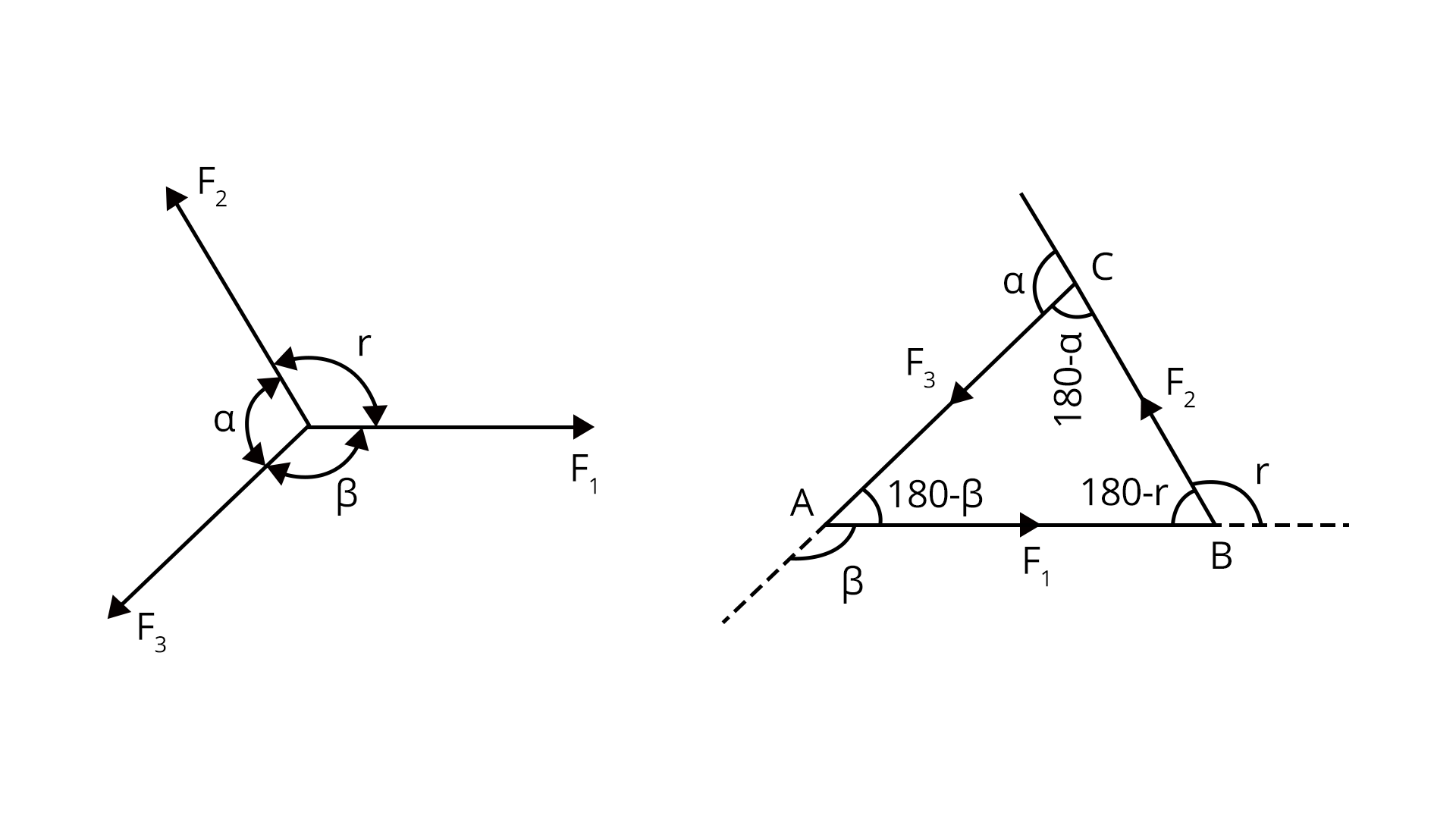Momentum and Impulse $\vec {P}= m v$$I=\int_{t_1}^{t_2}\vec {F} dt$ Law of conservation of momentum m1v1=m2v2 Miscellaneous formulae Bullets travelling with a velocity v hitting the wall:${F_{wall}}=n m v$n- Number of bulletsLiquid jet of area A moving with velocity v hits the wall:${F_{wall}}= 2 A {v^2} \cos \theta$ 7. Friction ${F_r}=\mu N$Kinetic friction Fk=k N ${F_r}={\mu_{k}} N$ 8. Centripetal Force $F= m {\omega^2}r$ 9. The apparent weight of the body in the lift (When lift moving in an upward direction) W= m (g+a)

### Solved Examples

1. An object of mass 0.2 kg is thrown vertically upwards. Calculate the magnitude of the net force acting on the body and direction,

1. During its upward motion

2. During its downward motion

3. At the highest point at which the object is considered to be at rest. Will your answers change if the object was thrown at an angle of 45° in the horizontal direction and ignoring the air resistance?

Sol:

Given,

Mass of the object=m=0.2 kg

Assume value of acceleration due to gravity=g=10 m/s2

1. During upward direction

When the object is thrown upward, the acceleration due to gravity g is acting downward, therefore, the net force is acting in a downward direction.

${F_{net}}=m g= 0.2\times 10= 2N$

1. During downward motion also the net force and direction remain the same i.e, F= 2N and the direction of force acting is downwards

2. The object will not be at rest when it reaches the highest point but it will have the horizontal component of velocity. Therefore, the direction and magnitude of the net force on the object will not change even if it is thrown at 45°.

Key point: Here only acceleration that acts will be acceleration due to gravity since the object was subjected to free fall.

2. A body of mass 10 kg is acted upon by two perpendicular forces 10 N and 12 N. Calculate the magnitude and direction of the acceleration of the body.

Sol:

Given,

The mass of the object=m=10 kg

Forces acting on the body F1= 10 N and F2=12 N

Now we are asked to determine the magnitude and direction of the body. As a first step, we must calculate the resultant force acting on the body.

$\vec {F_{net}}=\sqrt{{{F_1}^2}+{{F_2}^2}+2 {F_1}{F_2} \cos \theta (\theta=90^o)}$

$\vec {F_{net}}=\sqrt{{10^2}+{12^2}}=15.62\simeq17$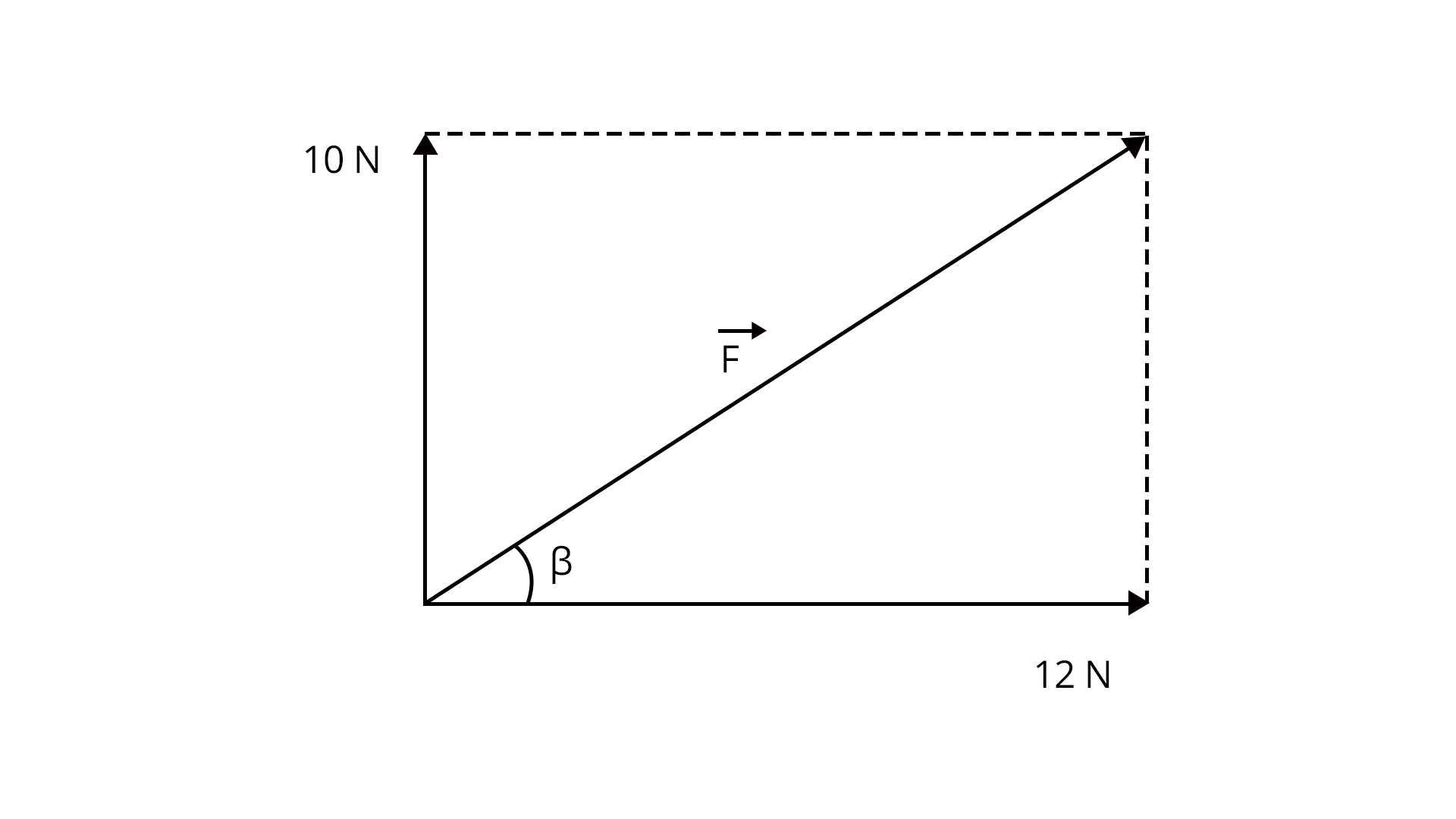The acceleration of the body,

$\vec {a}=\dfrac {\vec {F_{net}}}{m}=\dfrac {17}{10}=1.7 m/{s^2}$ in the same direction as the resultant force.

The direction of the acceleration is

$\tan \beta =\dfrac {10}{12}=0.8333$

$\beta =\tan^{-1}(0.833)= 39.7^o$ With 12 N force.

Key point: Formula for resultant force when two forces acting perpendicular to each other is $\vec {F_{net}}=\sqrt{{{F_1}^2}+{{F_2}^2}}$.

### Previous Year Questions from JEE Paper

1. A block of mass slides along a floor while a force of magnitude F is applied to it at an angle $\theta$ as shown in the figure. The coefficient of kinetic friction is ${\mu_k}$. Then, the block's acceleration 'a' is given by : (g is the acceleration due to gravity) (March -2021)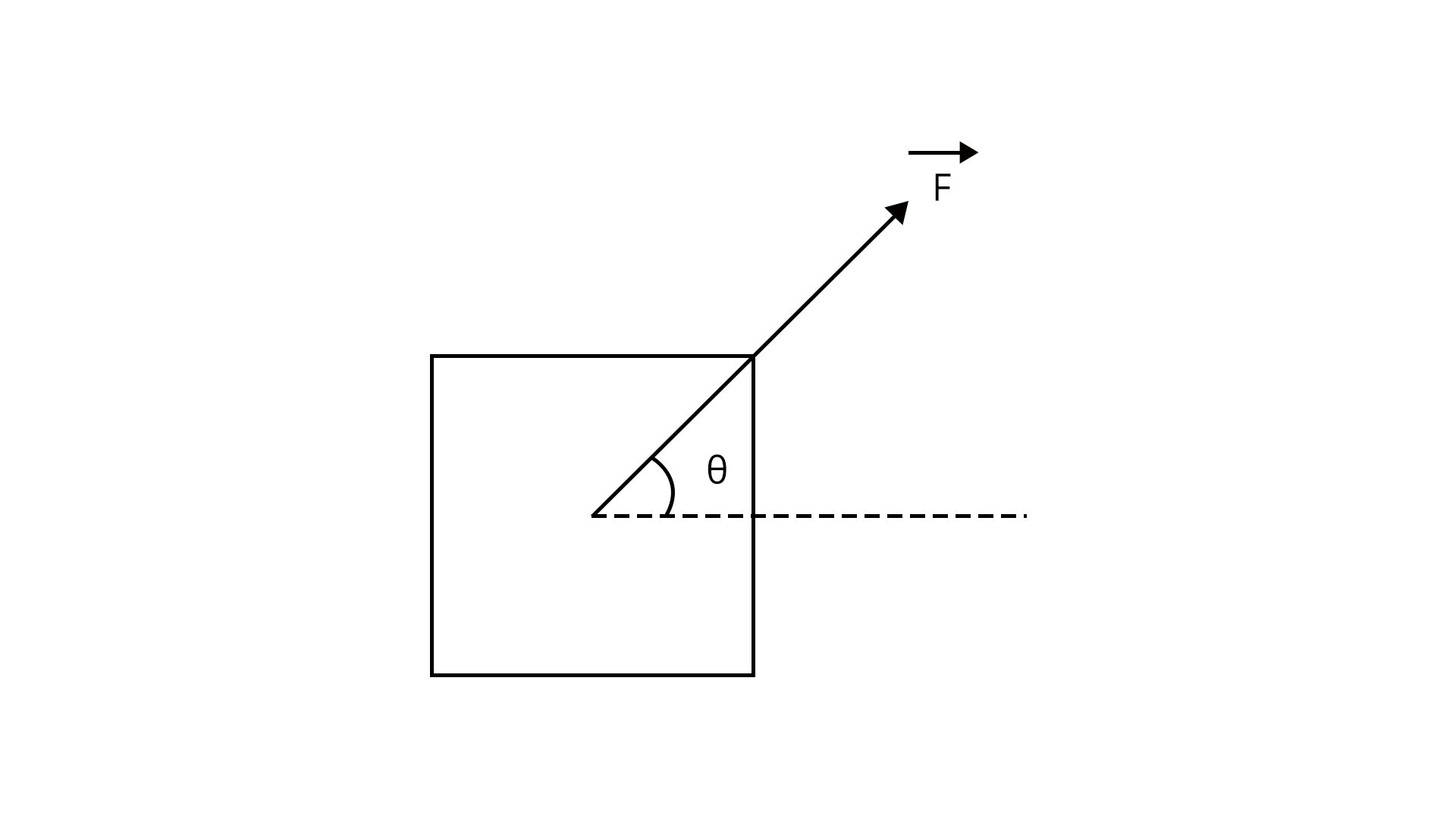Sol:

Given,

A block of mass ‘m’ is moving with the force F. We are asked to determine the acceleration of the block. This can be easily solved with the help of FBD. Let us draw the FBD for the object.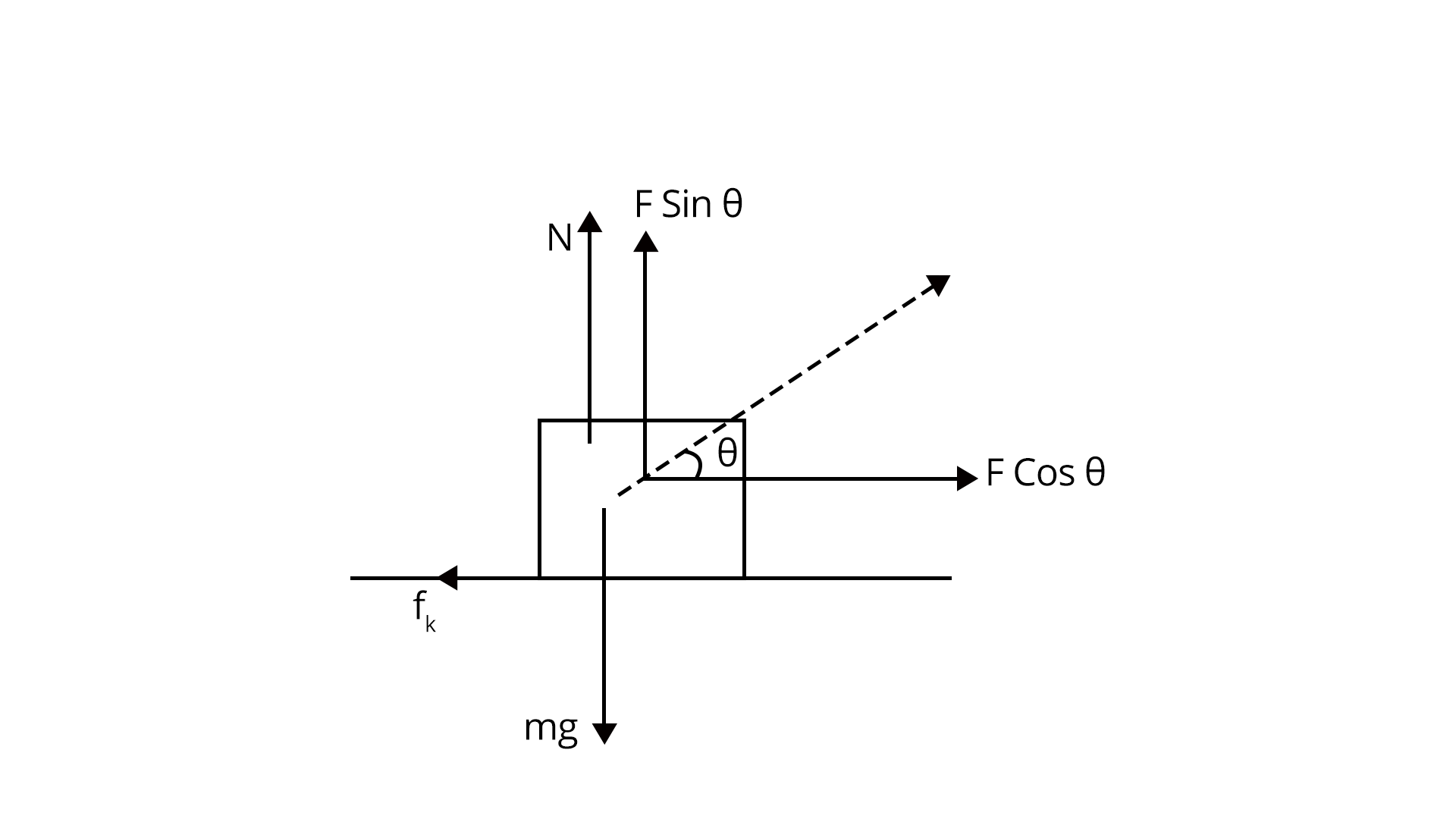From FBD we write:

$N+F \sin \theta=mg$

$\Rightarrow N=mg-F \sin \theta$

$F\cos \theta-{f_k}=ma$

$\Rightarrow F\cos \theta-{\mu_k}N=ma$

$\Rightarrow F\cos \theta-{\mu_k}(mg-F \sin \theta)=ma$

$\Rightarrow a=\dfrac {F}{m} \cos \theta -{\mu_k}(g-\dfrac {F}{m} \sin \theta)$

Trick: We can solve these problems just with the help of FBD, FBD should have all the forces acting on the body.

2. A block of mass 5 kg is (i) pushed in case (A) and (ii) pulled in case (B), by force F=20 N, making an angle of 300 with the horizontal, as shown in the figures. The coefficient of friction between the floor is $\mu = 0.2$. The difference between the accelerations of the block in case (B) and case (A) will be: (JEE-2019)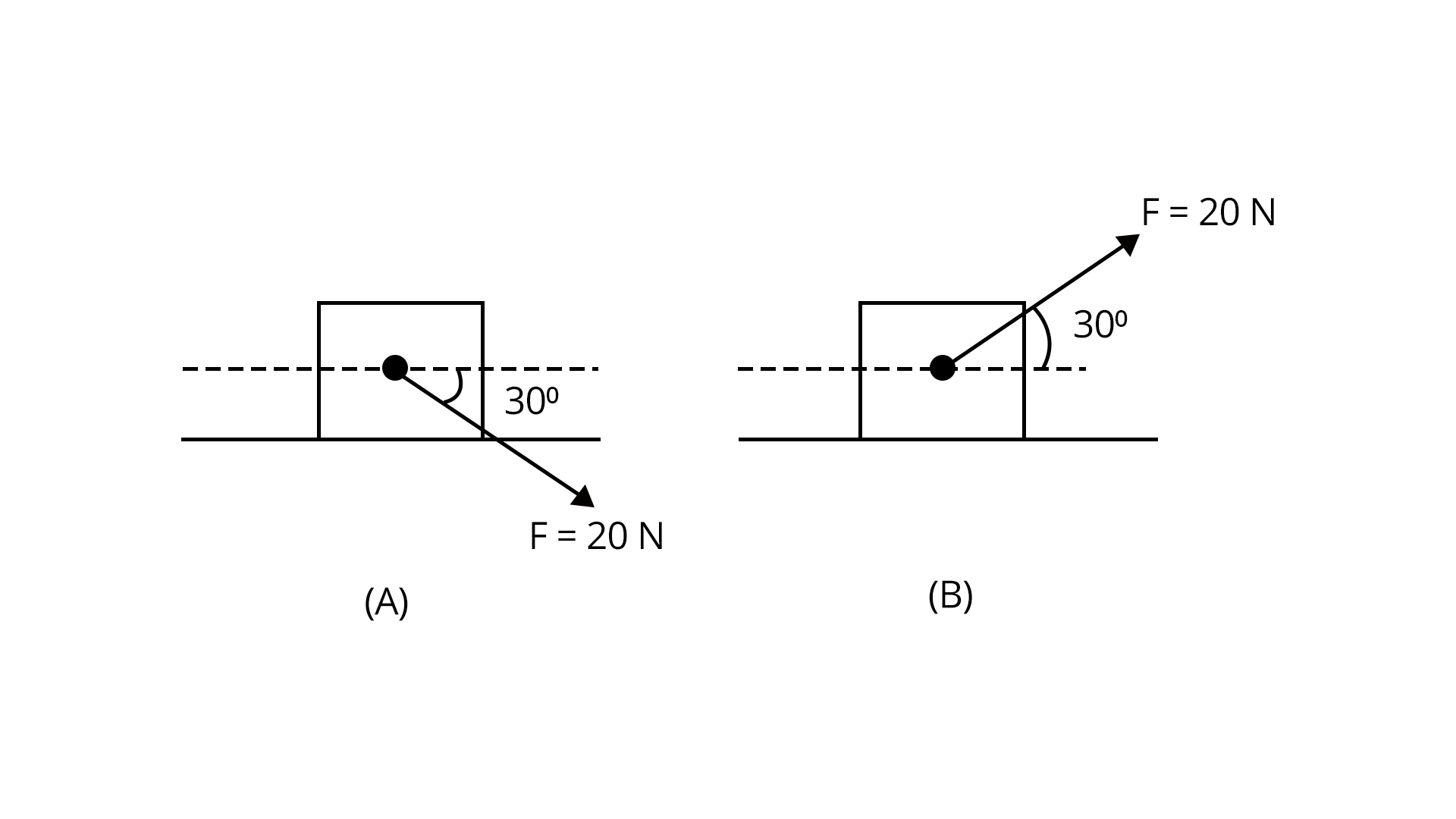Sol:

Given,

Mass of the block=m=5 kg

The magnitude of the force acting on the block=F=20 N

Case A:

In case A, the block is pushed over the surface.

Free body diagram of the block is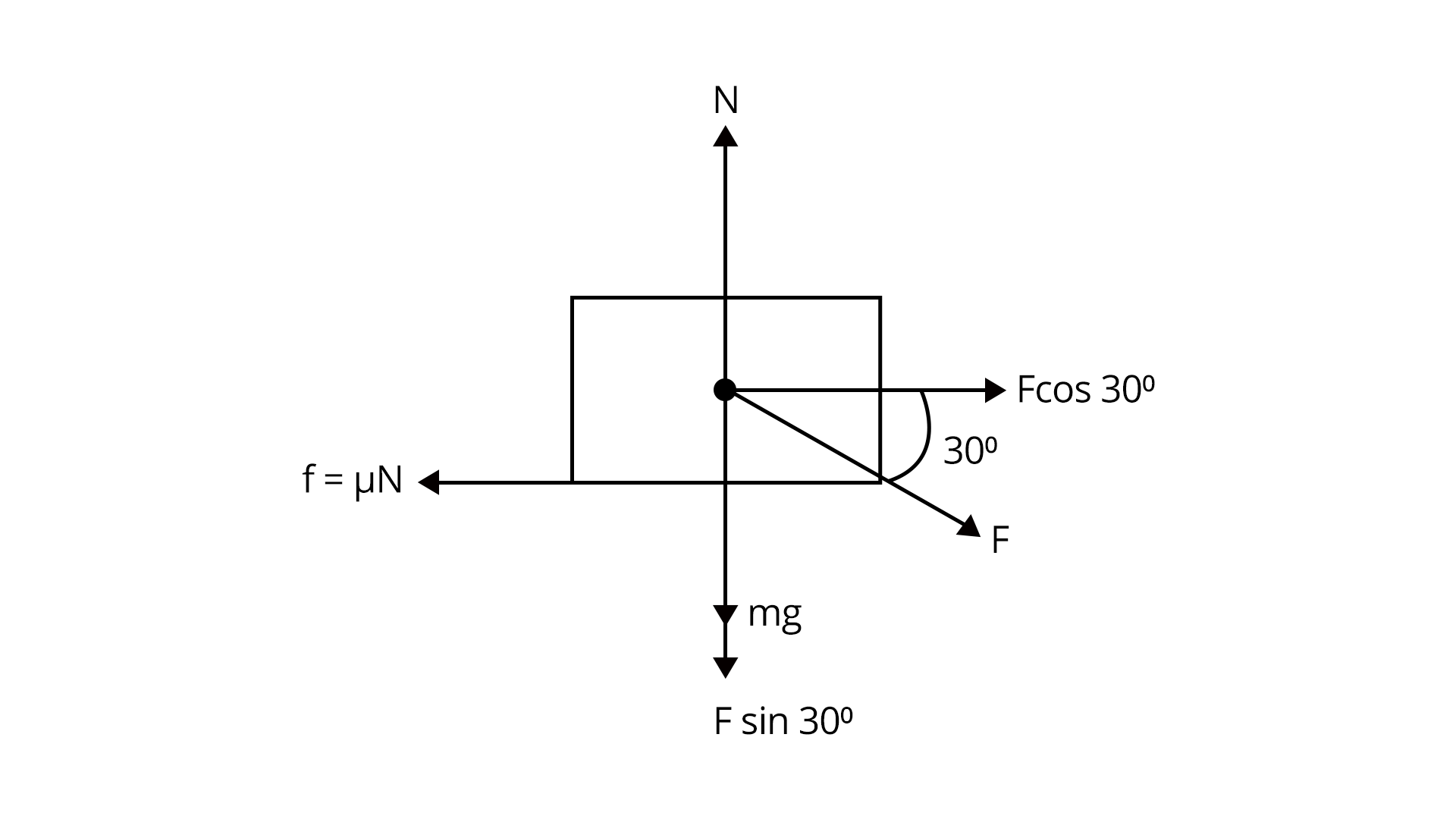From FBD we get,

${N_1}=mg+F \cos {30^o}$

${N_1}=50+ 20 (\dfrac {1}{2})=60 N$

Then the net acceleration in case A

$m {a_1}= F \cos {30^o}-{f}=20 \dfrac {\sqrt {3}}{2}-(0.2\times 60)=10 \sqrt{3}-12$

${a_1}=\dfrac {10 \sqrt{3}-12}{5}$

Case B: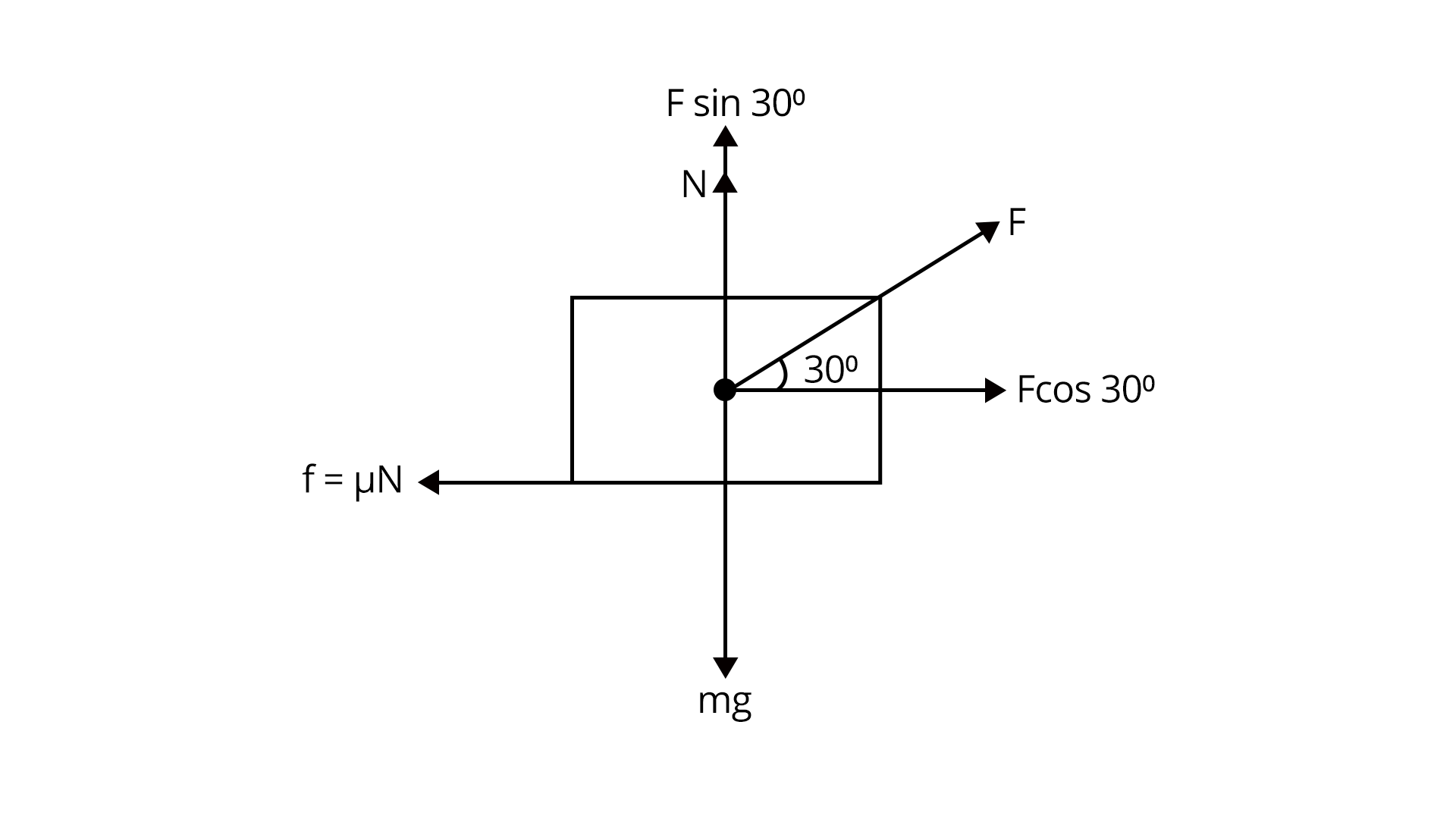From FBD, net force

Here,

$N+F \sin {30^o}=mg$

$N=mg-F \sin {30^o}$

${F_{net}}=F \cos {30^o}-{f}=10 \sqrt {3}-\mu N$

${F_{net}}=10 \sqrt {3}-\mu (mg-F \sin (30^o))=10\sqrt{3}-8$

$m{a_2}=10\sqrt{3}-8$

${a_2}=\dfrac {10\sqrt{3}-8}{5}$

Therefore,

${a_2}-{a_1}=0.8 m/{s^2}$

Trick: Resolving the given vector into components is important in order to solve the above example.

### Practice Questions

1. A block of mass 2kg rests on a rough inclined plane making an angle of 30° with the horizontal. The coefficient of static friction between the block and the plane is 0.7. The frictional force on the block is (Ans: 9.8 N)

2. A car is moving on a circular horizontal track of a radius of 10m with a constant speed of 10 m/s. A plumb bob is suspended from the roof of the car by a light rigid rod. The angle made by the rod with the track is (g = 10 m/s2)  (Ans: 450)

### Conclusion

In this article we have provided important information regarding the chapter laws of motion such as important concepts, formulae, etc.. Students should work on more solved examples and newton's laws examples for securing good grades in the JEE exams.

## Important Related Links for JEE Main 2022-23

See More## JEE Main Important Dates

View all JEE Main Exam Dates
JEE Main 2023 January and April Session exam dates and revised schedule have been announced by the NTA. JEE Main 2023 January and April Session will now be conducted on 24-Jan-2023 to 31-Jan-2023 and 6-Apr-2023 to 12-Apr-2023, and the exam registration closes on 12-Jan-2023 and Apr-2023. You can check the complete schedule on our site. Furthermore, you can check JEE Main 2023 dates for application, admit card, exam, answer key, result, counselling, etc along with other relevant information.
See More
View all JEE Main Exam Dates## JEE Main Information

Last updated date: 21st Mar 2023
Total views: 87.9k
Views today: 10

Application Form
Eligibility Criteria
Reservation Policy
Exam Centres
NTA has announced the JEE Main 2023 January session application form release date on the official website https://jeemain.nta.nic.in/. JEE Main 2023 January and April session Application Form is available on the official website for online registration. Besides JEE Main 2023 January and April session application form release date, learn about the application process, steps to fill the form, how to submit, exam date sheet etc online. Check our website for more details. April Session's details will be updated soon by NTA.## JEE Main Syllabus

View JEE Main Syllabus in Detail

Last updated date: 21st Mar 2023
Total views: 87.9k
Views today: 10

It is crucial for the the engineering aspirants to know and download the JEE Main 2023 syllabus PDF for Maths, Physics and Chemistry. Check JEE Main 2023 syllabus here along with the best books and strategies to prepare for the entrance exam. Download the JEE Main 2023 syllabus consolidated as per the latest NTA guidelines from Vedantu for free.
See More
View JEE Main Syllabus in Detail## JEE Main 2023 Study Material

View all study material for JEE Main
JEE Main 2023 Study Materials: Strengthen your fundamentals with exhaustive JEE Main Study Materials. It covers the entire JEE Main syllabus, DPP, PYP with ample objective and subjective solved problems. Free download of JEE Main study material for Physics, Chemistry and Maths are available on our website so that students can gear up their preparation for JEE Main exam 2023 with Vedantu right on time.
See More
All
Mathematics
Physics
Chemistry
See All## JEE Main Question Papers

see all
Download JEE Main Question Papers & ​Answer Keys of 2022, 2021, 2020, 2019, 2018 and 2017 PDFs. JEE Main Question Paper are provided language-wise along with their answer keys. We also offer JEE Main Sample Question Papers with Answer Keys for Physics, Chemistry and Maths solved by our expert teachers on Vedantu. Downloading the JEE Main Sample Question Papers with solutions will help the engineering aspirants to score high marks in the JEE Main examinations.
See MoreView all JEE Main Important Books

Last updated date: 21st Mar 2023
Total views: 87.9k
Views today: 10

In order to prepare for JEE Main 2023, candidates should know the list of important books i.e. RD Sharma Solutions, NCERT Solutions, RS Aggarwal Solutions, HC Verma books and RS Aggarwal Solutions. They will find the high quality readymade solutions of these books on Vedantu. These books will help them in order to prepare well for the JEE Main 2023 exam so that they can grab the top rank in the all India entrance exam.
See More
Maths
NCERT Book for Class 12 Maths
Physics
NCERT Book for Class 12 Physics
Chemistry
NCERT Book for Class 12 Chemistry
Physics
H. C. Verma Solutions
Maths
R. D. Sharma Solutions
Maths
R.S. Aggarwal Solutions
See All## JEE Main Mock Tests

View all mock tests
JEE Main 2023 free online mock test series for exam preparation are available on the Vedantu website for free download. Practising these mock test papers of Physics, Chemistry and Maths prepared by expert teachers at Vedantu will help you to boost your confidence to face the JEE Main 2023 examination without any worries. The JEE Main test series for Physics, Chemistry and Maths that is based on the latest syllabus of JEE Main and also the Previous Year Question Papers.
See More## JEE Main 2023 Cut-Off

JEE Main Cut Off

Last updated date: 21st Mar 2023
Total views: 87.9k
Views today: 10

NTA is responsible for the release of the JEE Main 2023 January and April Session cut off score. The qualifying percentile score might remain the same for different categories. According to the latest trends, the expected cut off mark for JEE Main 2023 January and April Session is 50% for general category candidates, 45% for physically challenged candidates, and 40% for candidates from reserved categories. For the general category, JEE Main qualifying marks for 2021 ranged from 87.8992241 for general-category, while for OBC/SC/ST categories, they ranged from 68.0234447 for OBC, 46.8825338 for SC and 34.6728999 for ST category.
See More## JEE Main 2023 Results

Last updated date: 21st Mar 2023
Total views: 87.9k
Views today: 10

NTA will release the JEE Main 2023 January and April sessions exam dates on the official website, i.e. {official-website}. Candidates can directly check the date sheet on the official website or https://jeemain.nta.nic.in/. JEE Main 2023 January and April sessions is expected to be held in February and May. Visit our website to keep updates of the respective important events of the national entrance exam.
See More
Rank List
Counselling
Cutoff
JEE Main 2023 state rank lists will be released by the state counselling committees for admissions to the 85% state quota and to all seats in IIT colleges. JEE Main 2023 state rank lists are based on the marks obtained in entrance exams. Candidates can check the JEE Main 2023 state rank list on the official website or on our site.## JEE Top Colleges

View all JEE Main 2023 Top Colleges

Last updated date: 21st Mar 2023
Total views: 87.9k
Views today: 10

Want to know which Engineering colleges in India accept the JEE Main 2023 scores for admission to Engineering? Find the list of Engineering colleges accepting JEE Main scores in India, compiled by Vedantu. There are 1622 Colleges that are accepting JEE Main. Also find more details on Fees, Ranking, Admission, and Placement.
See More## FAQs on JEE Important Chapter - Laws of Motion

FAQ

1. What is the weightage of the laws of motion in JEE?

Nearly 3-4 questions arise in the exam from this chapter covering about 10 marks which makes about 4% of the total marks.

2. What are the key points that need to be practised for solving questions from laws of motion?

Students should practice how to draw the free body diagram for solving the questions from the laws of motion.

3. Are previous year's questions enough for JEE Main?

To score 200+ in MAIN, NCERT (both 11th and 12th) and previous year JEE Main papers are sufficient. Solving the previous 10-year IIT-JEE examinations offers us a tremendous advantage because 3 to 4 questions with identical alternatives are guaranteed to be repeated every year.## JEE Main Upcoming Dates

Vedantu offers free live Master Classes for CBSE Class 6 to 12, ICSE, JEE Main, JEE 2023, & more by India’s best teachers. Learn all the important concepts concisely along with amazing tricks to score high marks in your class and other competitive exams.
See MoreJEE Main Exam April Session Starts
06 May 202345 days remainingJEE Main Exam April Session Ends
12 May 202352 days remaining## Notice board

JEE News
JEE BlogsTrending pages